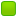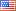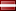###Author Topic: Check if remainder  (Read 2499 times)

####IronCanTaco

• Jr. Member
•• Posts: 7##### Check if remainder
« on: November 22, 2013, 10:41:59 PM »
So as the tittle already states, I would like to check remainder when dividing two numbers.

This is pretty basic stuff in C++, but how to do this in NASM?
I know how to read input, making loop etc so I just need some pointers on how to use div instruction.

####Frank Kotler

• NASM Developer
• Hero Member
•• Posts: 2461
• Country:##### Re: Check if remainder
« Reply #1 on: November 23, 2013, 02:01:06 AM »

"div" is tricky in that the operands you don't see depend on the one operand you provide. Dividing by a byte...
Code: [Select]
`div bl; ordiv byte [somenumber]`divides ax by bl (or somenumber) and puts the quotient in al and the remainder in ah. If the quotient won't fit in al (if ah >= bl), it raises an exception. This exception is sometimes reported as "divide by zero error" or "floating point error". Very helpful! (not) Chances are, you want zero in ah before the "div". Some other sane value - less than bl - is okay, but remember that the CPU cares what's in ah, even if you don't. After the "div", the remainder is in ah. Check it any way you like - "cmp ah, 0", "test ah, ah", "or ah, ah"...

Dividing by a word is similar. "div" divides dx:ax (dx * 64k + ax) by bx (or whatever) and puts the quotient in ax and the remainder in dx. Again, if the quotient won't fit in ax - exception! Check dx for the remainder.

By a dword, "div" divides edx:eax (edx * 4G + eax) by ebx (or whatever) and puts the quotient in eax and the remainder in edx. As you're probably beginning to suspect, if it won't fit, go boom.

I ASSume it's the same deal dividing a 128-bit number  by a 64-bit number, but I haven't tried it. Takes too long to check the result.So the "trick" is to pay attention to what "div" is dividing by what. The registers you want to be zero (or some sane value) before the "div" are the same ones you'll be checking after the "div" for the remainder - ah, dx, edx... rdx? Depending on how big your numbers are...

Best,
Frank

####encryptor256

• Full Member
•• Posts: 250
• Country:• Win64 .##### Re: Check if remainder
« Reply #2 on: November 23, 2013, 07:59:27 AM »
Hi!

Tested example code:
Code: [Select]
`xor rdx,rdxmov rax,10mov rcx,7div rcx `After division:
Result, rax is 1, rdx is 3.

Bye, Encryptor256!
Encryptor256's Investigation \ Research Department.